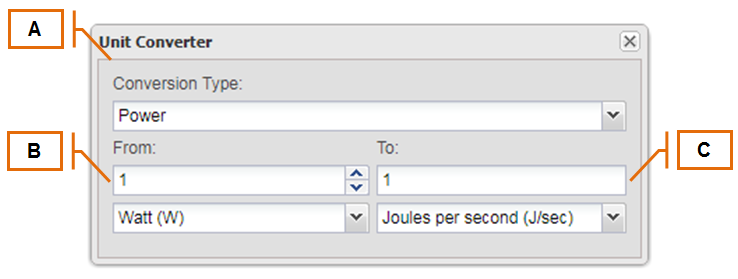# MyEyedro User Guide v4

### 7.1.Unit Converter-v4#Field How It’s Used A Conversion Type Select the type of measurement you’d like to use in the conversion. Choosing the type will change the list of available units: Power: Watt (W), Kilowatt (kW), Megawatt (MW), Joules per second (J/sec), Joules per hour (J/h), Calories per second (cal/sec), Calories per hour (cal/h), BTUs per second (Btu/sec), BTUs per hour (Btu/h), Ton [refrigeration] (RT), Horsepower [mechanical] (hp), Horsepower [electric] (hp), Horsepower [metric] (hp)Energy: Watt Hour (Wh), Kilowatt Hour (kWh), Megawatt Hour (MWh), Joule (J), Kilojoule (kJ), Megajoule (MJ), Calorie (cal), Kilocalorie (kcal), British Thermal Unit (Btu), Thousand Btu (MBtu), Million Btu (MMBtu), Tonne of Oil Equivalent (toe)Temperature: Celsius (C°), Fahrenheit (°F), Kelvin (°K) B Convert From Enter the value to be converted and select the appropriate starting unit. C Result Selecting the conversion unit will automatically populate the result field with the calculated value of the conversion.
Yes NoSuggest edit
Last updated on 16-May-2022
0 of 0 users found this section helpful
Suggest Edit